# System Software Unix IPC API

## Contents

Low level I/O
Pipes
Shared memory
A queue using an array
Implementing a pipe using a queue
Why it is wrong

## Low level I/O

This is revision material. I/O is done using block reads and writes. Files must be opened before reading or writing, and should be closed at the end. Opening a file returns a file descriptor which is an integer. This integer is an index into the table of files that are open for a process. Typical statements to read and write using this block I/O are
```#define SIZE 1024
char buf[SIZE];

src = open(infile, O_RDONLY);
dst = creat(outfile, 0777);
> 0)
```

## Pipes

A pipe is used for one-way communication of a stream of bytes. The command to create a pipe is pipe(), which takes an array of two integers. It fills in the array with two file descriptors that can be used for low-level I/O.

### Creating a pipe

```int pfd;

pipe(pfd);
```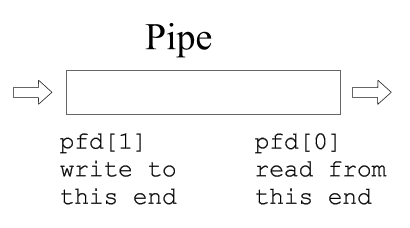### I/O with a pipe

These two file descriptors can be used for block I/O
```write(pfd, buf, size);
```

### Fork and a pipe

A single process would not use a pipe. They are used when two processes wish to communicate in a one-way fashion. A process splits in two using fork(). A pipe opened before the fork becomes shared between the two processes.

Before fork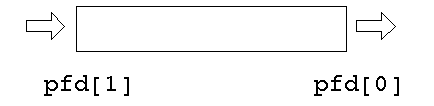After fork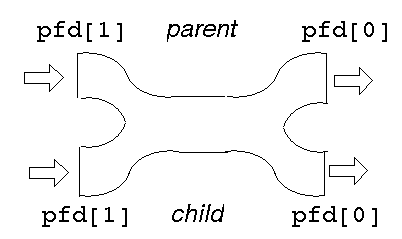This gives two read ends and two write ends. The read end of the pipe will not be closed until both of the read ends are closed, and the write end will not be closed until both the write ends are closed.

Either process can write into the pipe, and either can read from it. Which process will get what is not known.

For predictable behaviour, one of the processes must close its read end, and the other must close its write end. Then it will become a simple pipeline again.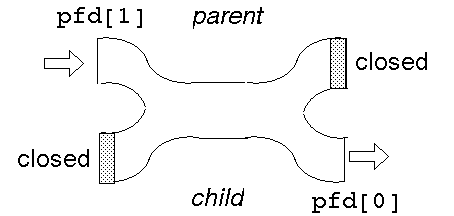Suppose the parent wants to write down a pipeline to a child. The parent closes its read end, and writes into the other end. The child closes its write end and reads from the other end.

When the processes have ceased communication, the parent closes its write end. This means that the child gets eof on its next read, and it can close its read end.

```#include <stdio.h>

#define SIZE 1024

int main(int argc, char **argv)
{
int pfd;
int pid;
char buf[SIZE];

if (pipe(pfd) == -1)
{
perror("pipe failed");
exit(1);
}
if ((pid = fork()) < 0)
{
perror("fork failed");
exit(2);
}

if (pid == 0)
{
/* child */
close(pfd);
!= 0)
close(pfd);
} else {
/* parent */
close(pfd);
strcpy(buf, "hello...");
/* include null terminator in write */
write(pfd, buf,
strlen(buf)+1);
close(pfd);
}
exit(0);
}

```

### dup

A pipeline works because the two processes know the file descriptor of each end of the pipe. Each process has a stdin (0), a stdout (1) and a stderr (2). The file descriptors will depend on which other files have been opened, but could be 3 and 4, say.

Suppose one of the processes replaces itself by an ``exec''. The new process will have files for descriptors 0, 1, 2, 3 and 4 open. How will it know which are the ones belonging to the pipe? It can't.

### Example:

To implement ``ls | wc'' the shell will have created a pipe and then forked. The parent will exec to be replaced by ``ls'', and the child will exec to be replaced by ``wc'' The write end of the pipe may be descriptor 3 and the read end may be descriptor 4. ``ls'' normally writes to 1 and ``wc'' normally reads from 0. How do these get matched up?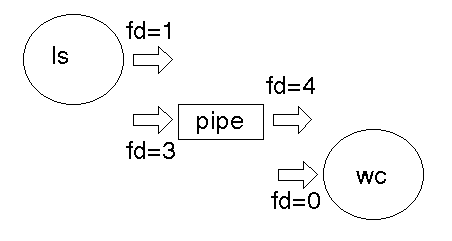The dup2() function call takes an existing file descriptor, and another one that it ``would like to be''. Here, fd=3 would also like to be 1, and fd=4 would like to be 0. So we dup2 fd=3 as 1, and dup2 fd=4 as 0. Then the old fd=3 and fd=4 can be closed as they are no longer needed.

After dup2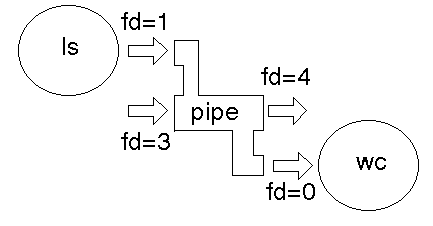After close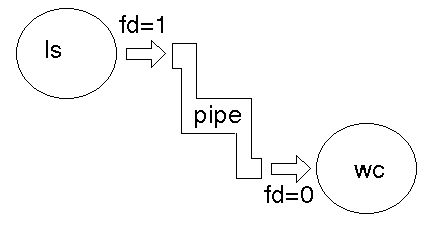Without any error checks, the program to do this is

```int main(void)
{
int pfd;

pipe(pfd);
if (fork() == 0) {
close(pfd);
dup2(pfd, 0);
close(pfd);
execlp("wc", "wc",
(char *) 0);
} else {
close(pfd);
dup2(pfd, 1);
close(pfd);
execlp("ls", "ls",
(char *) 0);
}
}
```
With checks, it is
```#include <stdio.h>

int main(void)
{
int pfd;
int pid;

if (pipe(pfd) == -1)
{
perror("pipe failed");
exit(1);
}
if ((pid = fork()) < 0)
{
perror("fork failed");
exit(2);
}
if (pid == 0)
{
close(pfd);
dup2(pfd, 0);
close(pfd);
execlp("wc", "wc",
(char *) 0);
perror("wc failed");
exit(3);
} else {
close(pfd);
dup2(pfd, 1);
close(pfd);
execlp("ls", "ls",
(char *) 0);
perror("ls failed");
exit(4);
}
exit(0);
}

```

### Variations

Some common variations on this method of IPC are:
1. A pipeline may consist of three or more process (such as a C version of ` ps | sed 1d | wc -l `). In this case there are lots of choices
1. The parent can fork twice to give two children.
2. The parent can fork once and the child can fork once, giving a parent, child and grandchild.
3. The parent can create two pipes before any forking. After a fork there will then be a total of 8 ends open (2 processes * two ends * 2 pipes). Most of these wil have to be closed to ensure that there ends up only one read and only one write end.
4. As many ends as possible of a pipe may be closed before a fork. This minimises the number of closes that have to be done after forking.
2. A process may want to both write to and read from a child. In this case it creates two pipes. One of these is used by the parent for writing and by the child for reading. The other is used by the child for writing and the parent for reading.

## Shared memory

Shared memory is when a single piece of memory is in the address space of two or more processes. If one process makes a change to this memory then it is changed for both processes.

The Linux shared memory calls come from Unix System V and are not yet standardised under POSIX. They are likely to be similar to these:

```#include <sys/types.h>
#include <sys/ipc.h>
#include <sys/shm.h>

int shmget(key_t key, int size,
int shmflg);
char *shmat(int shmid,
int shmflg);
```
The purpose of shmget is to create or to locate a piece of shared memory. The pieces of shared memory are identified by a key, which is an integer agreed upon by all processes that are going to use this piece of memory. The call also specifies the size of the memory and the permission flags (eg -rwx------).

The call shmat takes the shared memory identifier and performs a mapping of that into the address space of the process, returning a pointer to it. From then on it can be used just like any pointer to memory.

The call shmdt detaches it from the address space of the process.

So shmget and shmat are the shared memory equivalent of malloc, and shmdt is the equivalent of free.

### Example:

This program forks, creates shared memory on one side and just uses it on the other. The creating side writes the alphabet into this and the other side reads it.
```#include <sys/types.h>
#include <sys/ipc.h>
#include <sys/shm.h>

int main(void)
{
int shmid;
char *shmPtr;
int n;

if (fork() == 0) {

shmid = shmget(2041, 32,
0666 | IPC_CREAT);
if (shmid == -1)
exit(1);
shmPtr = shmat(shmid, 0,
0);
if (shmPtr == (char *) -1)
exit(2);
for (n = 0; n < 26; n++)
shmPtr[n] = 'a' + n;
for (n = 0; n < 26; n++)
putchar(shmPtr[n]);
putchar('\n');
+
} else {
/* wait for the child to terminate
so that we know it has written
to shared memory
*/
wait(NULL);
shmid = shmget(2041, 32,
0);
if (shmid == -1)
exit(1);
shmPtr = shmat(shmid, 0,
0);
if (shmPtr == (char *) -1)
exit(2);
for (n = 0; n < 26; n++)
putchar(shmPtr[n]);
putchar('\n');

shmdt(shmid);
}
exit(0);
}

```

## A queue using an array

A queue can be implemented using a ``wrap around'' array
```typedef queue {
int front;
int end;
int empty;
char elmts[SIZE];
}

init_queue(queue *q)
{
q->empty = 1;
}

int empty(queue *q)
{
return q->empty;
}

int full(queue *q)
{
if ((q->front + 1) % SIZE
== q->end)
return 1;
else return 0;
}

{
if (full(q))
return 0;
if (q->empty) {
q->empty = 0;
q->front = q->end = 0;
}
else
q->front = (q->front + 1) % SIZE;
q->elmts[q->front] = ch;
return 1;
}

int remove (queue q*, char *ch)
{
if (q->empty)
return 0;
*ch = q->elmts[q->front];
if (q->front == q->end)
q->empty = 1;
else
q->front = (q->front + 1) %
SIZE;
return 1;
}
```

## Implementing a pipe using a queue

It usually isn't, but perhaps it could be done this way (NB: this is pseudocode with missing details, not C)
```pipe()
{
shmget(1k)
q = shmat()
pfd = q
pfd = q
}

write(q, ch)
{
if full(q)
block
}

{
if empty(q)
block
remove(q, ch)
wakeup any blocked writer
}
```

## Why it is wrong

Consider this execution flow: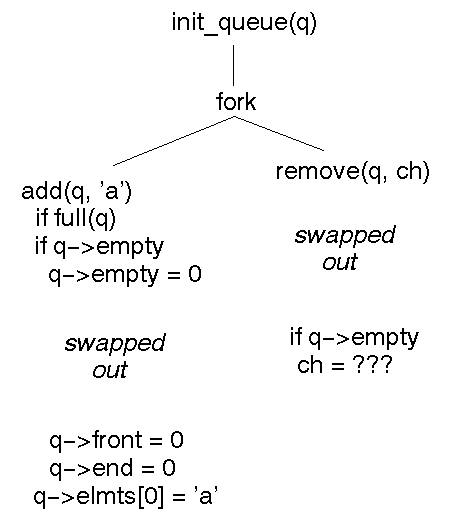The remove gets garbage because the add has not completed properly before being suspended.

Code that works correctly in a single sequential process may break when run in a shared memory situation by different processes.

To combat this, there are a variety of software and hardware methods. These in turn give rise to other problems, so what looks simple turns out to be quite complex.Systems Software Home

Jan Newmarch (http://jan.newmarch.name) <jan@newmarch.name>Copyright © Jan Newmarch under the terms of the Creative Commons Attribution 2.1 Australia License.